# DNA faculty working on numerics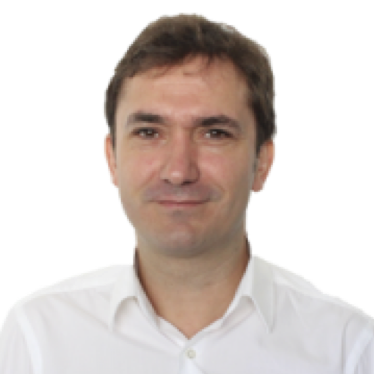Kurusch Ebrahimi-Fard's research explores the interface between applied and pure mathematics. His research fields include numerical integration of ordinary and stochastic differential equations, rough path theory, free probability theory, nonlinear control theory, and perturbative quantum field theory. He is particularly interested in Hopf and Lie algebraic structures on graphs, partitions and other combinatorial objects in the context of the aforementioned fields.Jørgen Endal is interested in nonlinear equations of hyperbolic and parabolic type. Such equations include for example a transport term and a diffusion term. In particular, he is working on cases where the diffusion can be nonlocal. He has been treating numerical schemes for equations of such nonlocal nature, where convergence and error estimates are some of the key issues.Helge Holden studies numerical methods for nonlinear partial differential equations where one can prove that the methods converge to the correct solution. This includes the front-tracking method for hyperbolic conservation laws, and special finite difference methods for the Korteweg–de Vries equation and the Benjamin–Ono equation. In addition, Holden works in collaboration with SINTEF on numerical methods for flow of hydrocarbons in porous media.Espen R. Jakobsen is working on numerical methods for different classes of nonlinear partial differential equations and stochastic differential equations. The models studied include diffusion, convection-diffusion, control theory, and stochastics and finance. Part of this research has been devoted to recent nonlocal or fractional models. Key questions are the design of robust methods and the analysis of convergence and error estimates for such methods.Trond Kvamsdal works on Computational Mathematics, i.e. development of new theories/methods within mathematics and numerical analysis to make robust and efficient algorithms for solving challenging (linear and non-linear) partial differential equations relevant within different areas of science and technology. The main area of research is adaptive isogeometric finite element methods for structural/solid and fluid mechanics.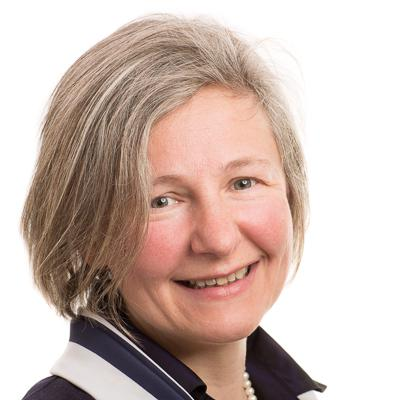Elena Celledoni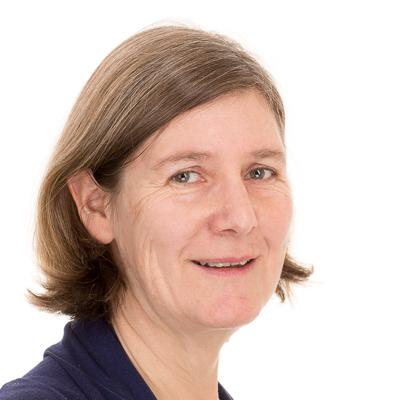Anne Kværnø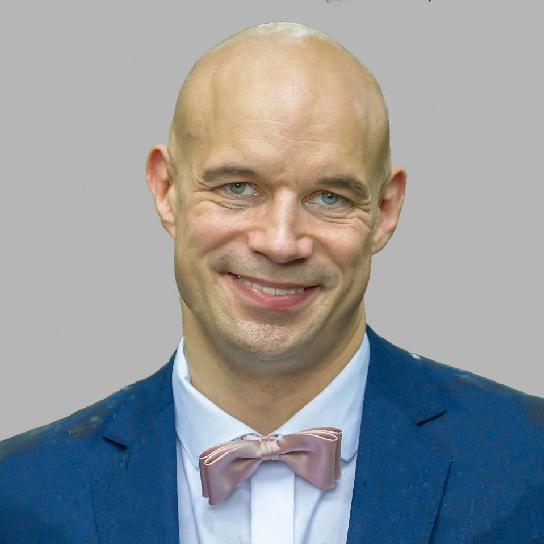Andre Massing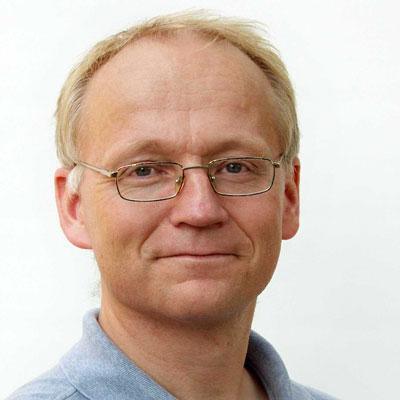Brynjulf Owren

## Numerics right barFrom the paper: E Celledoni, M. Eslitzbichler. Shape Analysis on Lie Groups with Applications in Computer Animation.